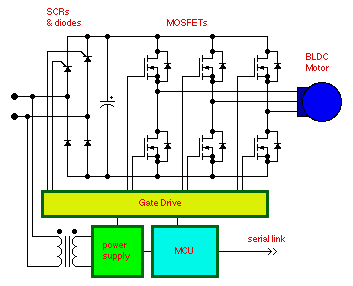• Introduction
• What is in this manual
• What is Caspoc
• User interface
• Introduction
• Starting
• Simulation
• Editing
• Viewing and printing
• Getting Started
• Basic editing
• Simulation in the time domain
• Basic User Interface Topics
• Editing
• Simulation
• Viewing
• Library
• Reports
• Project management
• Circuit and Block Diagram Components
• Introduction
• Cscript and user defined functions
• Component parameters
• Modeling Topics
• Introduction
• Power Electronics
• Semiconductors
• Electrical Machines
• Electrical drives
• Power Systems
• Mechanical Systems
• Thermal Systems
• Magnetic Circuits
• Green Energy
• Coupling to FEM
• Experimenter
• Analog hardware description language
• Embedded C code Export
• Coupling to Spice
• Small Signal Analysis
• Matlab coupling
• Tips and tricks
• Appendices

## Six-Step with SCR Input BridgeOne method for controlling a three phase motor is to use an SCR input bridge to control the dc link voltage. The dc link voltage is controlled using dc phase angle control. The three phase inverter is then driven using six-step commutation. The input bridge controls the voltage and the three phase inverter controls the output frequency.

This method can be used for AC induction, Permanent Magnet Synchronous Machines and Brushless DC motors. The output voltage is very good. However, the square output waveforms contain a large amount of odd harmonics. PWM control will provide lower harmonic distortion and less torque ripple.

Because the three phase bridge switches at low frequencies, MOSFETs or slow IGBTs may be used for the output bridge. Slow IGBTs are less expensive. MOSFETs can provide lower losses if money is no object.

This method may provide lower overall losses than using PWM. The switching losses for a PWM motor drive are considerable higher. However, the general trend is to use PWM.